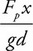# AP Physics 1 Question 129: Answer and Explanation

### Test Information

Question: 129

9.In the laboratory, a long platform of negligible mass is free to rotate on a fulcrum. A force probe is placed a fixed distance x from the fulcrum, supporting the platform. An object of fixed mass is placed a variable distance d from the fulcrum. For each position d, the force probe is read. It is desired to determine the mass of the object from a graph of data. Which of the following can determine the object’s mass?

• A. Plot the reading in the force probe times x on the vertical axis; plot the gravitational field times d on the horizontal axis. The mass is the slope of the line.
• B. Plot the reading in the force probe on the vertical axis; plot the distance d on the horizontal axis. The mass is the area under the graph.
• C. Plot the reading in the force probe on the vertical axis; plot the distance d multiplied by the distance x on the horizontal axis. The mass is the y-intercept of the graph.
• D. Plot the reading in the force probe times d on the vertical axis; plot the distance x on the horizontal axis. The mass is the slope of the line divided by the gravitational field.

A-Since the platform itself is of negligible mass, only two torques act on the platform: counterclockwise by the force of the force probe (FP), and clockwise by the downward force of the object (mg). Torque is force times distance from a fulcrum, so set these torques equal: FP(x) = mg(d). Solving for the mass, we get. Plot the numerator on the vertical, the denominator on the horizontal, and the slope is the mass m.